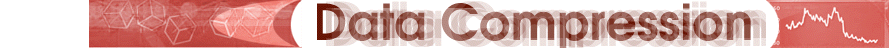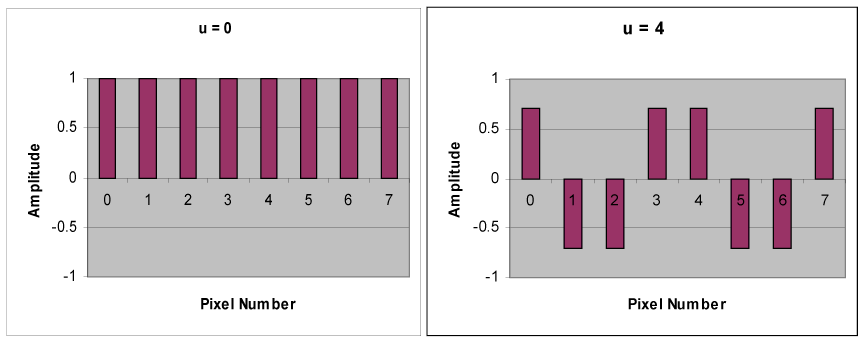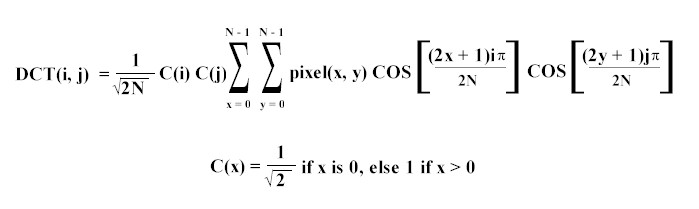Introduction
Algorithm
- DCT
- Coefficient Quantization
- Lossless Compression
Color
Future

The Discrete Cosine Transform (DCT)

The key to the JPEG baseline compression process is a mathematical transformation known as the Discrete Cosine Transform (DCT). The DCT is in a class of mathematical operations that includes the well known Fast Fourier Transform (FFT), as well as many others. The basic purpose of these operations is to take a signal and transform it from one type of representation to another. For example, an image is a two-dimensional signal that is perceived by the human visual system. The DCT can be used to convert the signal (spatial information) into numeric data ("frequency" or "spectral" information) so that the image’s information exists in a quantitative form that can be manipulated for compression.

The signal for a graphical image can be thought of as a three-dimensional signal. The X and Y-axes of the image’s signal are the two dimensions of the screen, while the amplitude of the signal, the Z-axis, is the value of the pixel at (X, Y). This can be represented visually by a two-dimensional array where each cell contains the numerical value of the pixel at that location. As the specifics of a two-dimensional DCT matrix are rather complex, we will simplify the problem by first considering the derivation and intentions of a one-dimensional DCT matrix.

The One-Dimensional DCTWe start with a set of eight arbitrary grayscale samples as charted below, where each bar represents the luminosity of a single pixel.

These values contain all the information necessary to define the eight pixels. Thus the ultimate goal is to compress this data so it can be stored or transmitted and later decompressed to reform the original image. However, as explained above, simple entropy or statistical encoding of this data will not be extremely effective because in continuous tone images, the levels of luminosity have equal probabilities of occurring. As a more effective alternative, the DCT can manipulate this data, separating information crucial to the definition of the image from information that’s presence is not perceivable by the human eye. The insignificant information can then be "discarded" through the quantization phase of JPEG coding, thus achieving large-scale compression. Simply put, the purpose of the DCT transformation phase is to identify "pieces of information in the image’s signal that can be effectively ‘thrown away’ without seriously compromising the quality of the image" (Nelson 359). No information is lost, nor is any compression achieved, in the DCT stage. This initial phase is merely a preparatory step that allows for and leads to the lossy coefficient quantization stage that follows.

The first step involved in the "rearrangement" of the data displayed in figure (a) is to perform a level shift by 128, the result of which is shown in figure (b) below.The samples have values in the range of 0 to 255. By shifting the level of their graph by 128, half their range, we obtain the values of f(x) in figure (b). Using f(x), we can decompose the eight sample values into a set of waveforms of different spatial frequencies. This decomposition is where the separation of the more significant low-frequency components from the less significant high-frequency components takes place.

Below is a set of waveforms of eight different spatial frequencies, all of uniform amplitude and each sampled at eight points.

Below is a set of cosine waveforms of eight different spatial frequencies.The top left waveform (u = 0) is simply a constant, whereas the other seven waveforms (u = 1, …, 7) show an alternating behavior at progressively higher frequencies. These waveforms, which are called cosine basis functions, are independent, meaning that there is no way that a given waveform can be represented by any combination of the other waveforms. However, the complete set of eight waveforms, when scaled by numbers called coefficients and added together, can be used to represent any eight sample values such as those in figure (b). The intention is to use the Discrete Cosine Transform to determine the values of the coefficients. The coefficients plotted in figure (c) below are the output of an 8-point DCT for the eight sample values in figure (b).There is a direct correlation between the magnitude of the coefficient for a given waveform and the impact of that particular waveform on the quality of the picture. The coefficient that scales the constant basis function (u = 0) is called the DC coefficient, while the other coefficients are called AC coefficients. Note that the DC term gives the average over the set of samples. Furthermore, the DC term is usually a great deal larger in magnitude than the AC terms, and, though it may not be evident in the small sampling depicted by figure (c), as the elements move farther away from the DC term, they tend to become lower and lower in value. Recall that the AC terms farther away from the DC term represent coefficients of waveforms of greater spatial frequencies. The fact that these coefficients tend to be smaller in magnitude suggests that higher-frequency image components play a relatively small role in the determining picture quality, while the majority of image definition comes from lower-frequency image components. This idea becomes extremely important when applied two-dimensionally to an image, for JPEG exploits this exact concept when deciding what information can be eliminated to achieve compression.

The Two-Dimensional DCT

The one-dimensional DCT described above can be extended to apply to two-dimensional image arrays. The two-dimensional cosine basis functions from which sample waveforms are composed are created by multiplying a horizontally oriented set of one-dimensional 8-point basis functions by a vertically oriented set of the same functions. It logically follows that the horizontally oriented set of basis functions represents horizontal frequencies and the other set of basis functions represents vertical frequencies.

By convention, the DC term of the horizontal basis functions is to the left, and the DC term for the vertical basis functions is at the top. Consequently, the top, left element of a two-dimensional DCT matrix contains a value that is almost always of a very great magnitude. Furthermore, mirroring the trend found in a one-dimensional DCT matrix, the farther away an AC term is from the DC term, the higher the frequency its corresponding waveform will have and the smaller its magnitude will be. Although the concept of the two-dimensional DCT is not as easily pictured using histograms as is the one-dimensional DCT, the two-dimensional version employs the same underlying principles and thus can be interpreted using identical reasoning (Pennebaker 33).

The actual formula for the two-dimensional DCT is shown below. The DCT is performed on an N x N square matrix of pixel values, and it yields an N x N square matrix of frequency coefficients. (In practice, N most often equals 8 because a larger block, though would probably give better compression, often takes a great deal of time to perform DCT calculations, creating an unreasonable tradeoff. As a result, DCT implementations typically break the image down into more manageable 8 x 8 blocks.) The DCT formula looks somewhat intimidating at first glance but can be implemented with a relatively straightforward piece of code.When writing code to implement this function, simple table lookups can replace several terms of the equation to simplify the appearance of the algorithm. The two cosine terms only need to be calculated once at the beginning of the program, and they can be stored for later use. Likewise, the C(x) terms can also be replaced with table lookups. Code to compute the DCT matrix for an N-by-N portion of a display looks somewhat like the following (adapted from The Data Compression Book by Mark Nelson).

for (i = 0; i < N; i++) {
for (j = 0; j < N; j++) {
temp = 0.0;
for (x = 0; x < N; x++) {
for (y = 0; y < N; y++) {
temp += Cosines[x][i] *
Cosines[y][j] *
Pixel[x][y];
}
}
temp *= sqrt(2 * N) * Coefficient[i][j];
DCT[i][j] = INT_ROUND(temp);
}
}

The above code is only one example of an algorithm that implements the DCT transformation on an N x N matrix. This particular algorithm is extremely inefficient due to a double nested for-loop. Although a considerably more efficient form of the DCT can be calculated using matrix operations, the algorithm displayed above is the most straightforward.

Below are two matrices representing the DCT input and DCT output blocks from a gray-scale image. Each element of the 8 pixel-by 8-pixel input matrix contains the value of the pixel at the corresponding (x, y) location. These integer values are fed to the DCT algorithm, creating the output matrix shown below it. Each element of the output matrix is a coefficient by which the waveform of the corresponding spatial frequency is multiplied in the decomposition of the image sample.

Input Pixel Matrix

 140 144 147 140 140 155 179 175 144 152 140 147 140 148 167 179 152 155 136 167 163 162 152 172 168 145 156 160 152 155 136 160 162 148 156 148 140 136 147 162 147 167 140 155 155 140 136 162 136 156 123 167 162 144 140 147 148 155 136 155 152 147 147 136

Output DCT Matrix

 186 -18 15 -9 23 -9 -14 19 21 -34 26 -9 -11 11 14 7 -10 -24 -2 6 -18 3 -20 -1 -8 -5 14 -15 -8 -3 -3 8 -3 10 8 1 -11 18 18 15 4 -2 -18 8 8 -4 1 -7 9 1 -3 4 -1 -7 -1 -2 0 -8 -2 2 1 4 -6 0

As shown in the output matrix, the DC coefficient, 186, is relatively large in magnitude, and the AC terms become lower in magnitude as they move farther from the DC coefficient. This means that by performing the DCT on the input data, we have concentrated the representation of the image in the upper left coefficients of the output matrix, with the lower right coefficients of the DCT matrix containing less useful information. The next step, quantization of the coefficients in the output matrix, "discards" the less useful data and in turn compresses the image data.